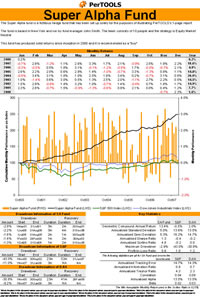Products: Quick search:CategoriesAreas / SectorsVendorsPlatformsFrom A to ZNew products My profile:My subscriptionMy shopping cartMy orders About Aertia:Who we areContact usLegal information

# PerTOOLS Financial Analysis for Excel

##### by Totally Un Limited Company

PerTOOLS Financial Analysis for Excel is an extremely valuable tool for Excel that allows anyone to easily analyse time-series data automatically, with flexibility, speed and full user control.Description | More information | Demos | Pricing | Sectors | PlatformsPerTOOLS Financial Analysis for Excel is an extremely valuable tool for Excel that allows anyone to easily analyse time-series data automatically, with flexibility, speed and full user control. You can choose from a range of over 50 functions (which includes an infinite number of implementations) each of which runs very quickly and can be called from a cell formula in exactly the same way you might use an Excel formula like =SUM() or =AVERAGE() All functions refresh automatically when data is added, deleted or changed and you can easily use thousands of these functions in a single spreadsheet without experiencing speed issues. All appropriate functions are supplied in both geometric and arithmetic versions so you can choose how to compound returns. A comprehensive manual is also provided which contains full explanations of all functions together with all necessary mathematical formulae and examples. An example spreadsheet is also provided which shows examples of all functions being used in practice. Full VBA integration is included. The functions have been written so that the user only needs to remember a minimum about how to use them. For example, the function =PT_CumRet_A() that computes an Arithmetically-Compounded Return can produce many different kinds of output depending upon the function inputs. It can produce: An output expressed at any frequency. For example, if the input returns are daily, the computed arithmetically-compounded return can be daily, weekly, monthly, annual or any other user-chosen frequency. This functionality is called UpScaling  An output for the whole period. In this case, this would be an arithmetically-compounded return expressed over the whole period of the input data The advantage is that the user doesn’t need to remember many different function names; he just needs to remember one. In this way, fewer functions can have many implementations. All of the functions, manual and example spreadsheet are included for one price. The new UpScaling functionality allows the user to compute any of the PerTOOLS statistics over any period. For example, suppose the user wanted to compute a Sharpe Ratio. No matter what the frequency of the input data is (daily weekly, monthly etc.), the user can compute a daily, weekly, monthly or annual Sharpe Ratio Available functions include: Arithmetically Compounded Return Geometrically Compounded Return Arithmetic Sharpe Ratio Geometric Sharpe Ratio Arithmetic Relative Sharpe Ratio Geometric Relative Sharpe Ratio Standard Deviation Gain Deviation Loss Deviation Downside Deviation Semi Deviation Maximum Drawdown Drawdown Analysis Maximum Run-Up Run-Up Analysis Alpha Beta Correlation R-Squared Skew Kurtosis Arithmetic Average Gain Geometric Average Gain Arithmetic Average Loss Geometric Average Loss Information Ratio Tracking Error Sortino Ratio Arithmetic Treynor Ratio Geometric Treynor Ratio Jensen's Alpha Up-Percentage Ratio Down-Percentage Ratio Percent-Gain Ratio Percent-Loss Ratio Up Capture Down Capture Up-Number Ratio Down-Number Ratio Gain-to-Loss Ratio Profit-to-Loss Ratio All functions Uses There are literally hundreds of uses for this product which include: Create custom statistics in Excel that update each month when new data is added Monitor peer-group performance Create custom functions of your own, easily Create custom reports branded with the company logo Create statistics for presentations. These statistics will update automatically each month when new data is added Integrate different data sources such as Reuters and Bloomberg and create your own custom analytics based on this data Analyse data from a database such as Pertrac and have the flexibility to compute statistics based on this data, easily and quickly by using Excel and the PerTOOLS functions Speed up VBA development times by using these functions within VBA Use in conjunction with PerTOOLS Automatic Data Extractor for Excel to add a whole new dimension to your analysis of Pertrac data Full instructions are provided in a detailed user-guide.ACTIONS » Send to a friend » Print this page » Subscribe to this product » See price list » See products from Totally Un Limited Company » Request a demo